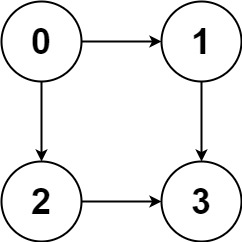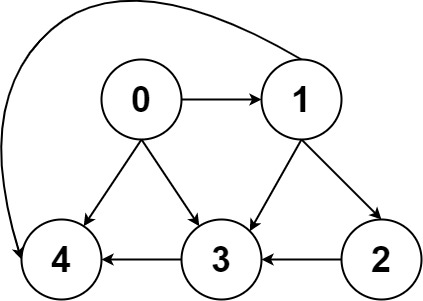1. 图的逻辑结构

# 图的逻辑结构

## 逻辑结构

N叉树的逻辑结构

``````/* 基本的 N 叉树节点 */
class TreeNode {
int val;
TreeNode[] children;
}
``````

``````/* 图节点的逻辑结构 */
class Vertex {
int id;
Vertex[] neighbors;
}
``````

• 邻接表很直观，我把每个节点 `x` 的邻居都存到一个列表里，然后把 `x` 和这个列表关联起来，这样就可以通过一个节点 `x` 找到它的所有相邻节点。
• 邻接矩阵则是一个二维布尔数组，我们权且称为 `matrix`，如果节点 `x``y` 是相连的，那么就把 `matrix[x][y]` 设为 `true`（上图中绿色的方格代表 `true`）。如果想找节点 `x` 的邻居，去扫一圈 `matrix[x][..]` 就行了。``````// 邻接表
// graph[x] 存储 x 的所有邻居节点
List<Integer>[] graph;

// 邻接矩阵
// matrix[x][y] 记录 x 是否有一条指向 y 的边
boolean[][] matrix;
``````

``````// 邻接矩阵
// graph[x] 存储 x 的所有邻居节点以及对应的权重
List<int[]>[] graph;

// 邻接矩阵
// matrix[x][y] 记录 x 指向 y 的边的权重，0 表示不相邻
int[][] matrix;
``````

## 图的遍历

``````/* 多叉树遍历框架 */
void traverse(TreeNode root) {
if (root == null) return;

for (TreeNode child : root.children) {
traverse(child);
}
}
``````

``````// 记录被遍历过的节点
boolean[] visited;
// 记录从起点到当前节点的路径
boolean[] onPath;

/* 图遍历框架 */
void traverse(Graph graph, int s) {
if (visited[s]) return;
// 经过节点 s，标记为已遍历
visited[s] = true;
// 做选择：标记节点 s 在路径上
onPath[s] = true;
for (int neighbor : graph.neighbors(s)) {
traverse(graph, neighbor);
}
// 撤销选择：节点 s 离开路径
onPath[s] = false;
}
``````## 上手题目``````输入：graph = [[1,2],,,[]]

````````````输入：graph = [[4,3,1],[3,2,4],,,[]]

``````

``````输入：graph = [,[]]

``````

``````输入：graph = [[1,2,3],,,[]]

``````

``````输入：graph = [[1,3],,,[]]

``````

• `n == graph.length`
• `2 <= n <= 15`
• `0 <= graph[i][j] < n`
• `graph[i][j] != i`（即，不存在自环）
• `graph[i]` 中的所有元素 互不相同
• 保证输入为 有向无环图（DAG）

``````//leetcode submit region begin(Prohibit modification and deletion)
class Solution {
// 记录所有路径

public List<List<Integer>> allPathsSourceTarget(int[][] graph) {
traverse(graph, 0, path);
return res;
}

void traverse(int[][] graph, int s, LinkedList<Integer> path) {
// 添加结点 s 到路径

int n = graph.length;
// 到达终点
if (s == n-1) {
path.removeLast();
return;
}

for (int v : graph[s]) {
traverse(graph, v, path);
}

// 从路径中移出结点 s
path.removeLast();
}
}
//leetcode submit region end(Prohibit modification and deletion)
``````

×